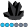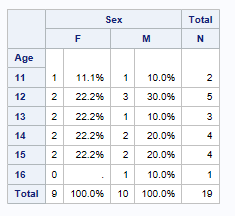PeteLund
Obsidian | Level 7

## Suppressing COLPCTN on PROC TABULATE total row

In PROC TABULATE, is there a way to suppress the COLPCTN value from the total (ALL) row?  Here's some simple code and the output...

``````proc format;
value MissingZero
. = '0'
other = [3.]
;
picture pcnt (round)
0 = ' - '
other = '009.9%'
;
quit;

proc tabulate data=sashelp.class missing;
class age sex;
table age all='Total', sex*(n=''*f=MissingZero. colpctn=''*f=pcnt.) all='Total';
run;``````I would like to not have the 100% on the total row - just the N.

Thanks.

## Re: Suppressing COLPCTN on PROC TABULATE total row

Hi:

If you know that the numbers will ALWAYS add up to exactly 100, you could change the picture format:

``````proc format;
value MissingZero
. = '0'
other = [3.]
;
picture pcnt (round)
0 = ' - '
100 = '   '
other = '009.9%'
;
quit;

proc tabulate data=sashelp.class missing;
title 'Tabulate';
class age sex;
table age all='Total', sex*(n=''*f=MissingZero. colpctn=''*f=pcnt.) all='Total';
run;
``````

Otherwise, I'd probably recommend moving to PROC REPORT because I suppose there could be a chance that your percents could add up to 99.99994 or something.

Cynthia

Discussion stats